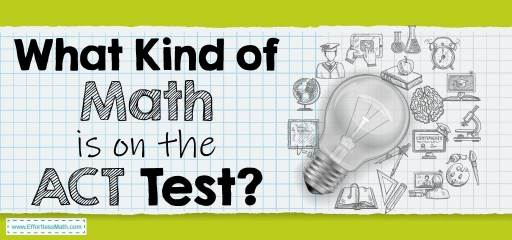# What Kind of Math Is on the ACT Test?ACT (the American College Testing Organization) is a standardized test that aims to assess students’ academic ability to be admitted to United States universities. The test is administered by a non-profit organization of the same name (ACT). The ACT was first introduced in November 1959 as a competitor to the SAT. ACT has since seen a gradual increase in the number of test-takers.

The ACT consists of four main sections: English, Reading, Mathematics, Science. The ACT also includes an optional 40-minute Writing Test.

The math section of the ACT consists of 60 multiple-choice questions that test takers have 60 minutes to answer. Test takers are also allowed to use personal calculators in the test math section.

Exam materials in the ACT Math section include the following:

• Pre-Algebra
• Elementary Algebra
• Intermediate Algebra
• Coordinate Geometry
• Plane Geometry
• Trigonometry

## Pre-Algebra

In this section, you are dealing with math terminology. Your knowledge of concepts such as integers, prime numbers will be tested. Zero rules, order of operations, manipulation of fractions, and decimals are other topics in this math part of the test.

## Elementary Algebra

In the Elementary Algebra part, your knowledge about inequalities, linear equations, ratios, percentages, and averages, which are basic concepts of algebra, is important.

## Intermediate Algebra

As the name implies, this section includes more complex concepts of algebra. To answer the questions in this section, you must master topics such as exponents, roots, and quadratic equations.

## Plane Geometry

To get points in this section, you must know geometric shapes, angles, perimeter, area, and volume well. You need to identify the similarities and make the necessary measurements using rules and relationships.

## Coordinate Geometry

Coordinate Geometry questions are about topics such as slope, distance, midpoint, parallel and perpendicular lines, points of intersection, and graphing. In this section, you should have information about basic graphing and finding points on the x, y-coordinate graph.

## Trigonometry

Trigonometry questions are very basic, which include trigonometric ratios and basic trigonometric identities. The basic functions of sine, cosine, and tangent are some of the topics in this section.

## FAQs:

### Is the ACT or SAT math harder?

The answer to this question is up to you. Neither test is harder than the other, but some test takers may find one test harder than the other.

### How do I study for the ACT Math?

How you prepare for the ACT Math test is very important. You should use the best prep books to help you prepare well for the ACT Math test. You can also use online practice tests for further practice after studying the ACT exam concepts.

### How is the ACT Test Scored?

In the ACT, each subject area is given a scaled score between 1 and 36. Then these area scores average your composite score, which is between 1 and 36. for more information, you can check here.

## More from Effortless Math for ACT Test …

### Are you looking for a FREE ACT Math course to help you prepare for your test?

Check out our Ultimate ACT Math Course.

Have a look at our comprehensive ACT Math Worksheets to help you practice and prepare for the ACT Math test.

### Looking for FREE ACT Math websites to find free online resources?

Here is our complete list of Top 10 Free Websites for ACT Math Preparation.

Have a look at our Full-Length ACT Math Practice Test and Free ACT Math Practice Test.

### Do you know the key differences between SAT Math and ACT Math?

Find your answer here: SAT Math vs. ACT Math: the key differences.

## Have any questions about the ACT Test?

### What people say about "What Kind of Math Is on the ACT Test? - Effortless Math: We Help Students Learn to LOVE Mathematics"?

No one replied yet.

X
52% OFF

Limited time only!

Save Over 52%

SAVE \$40

It was \$76.99 now it is \$36.99